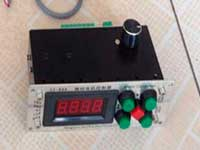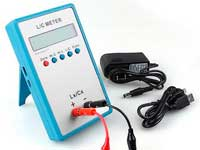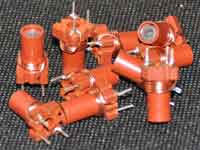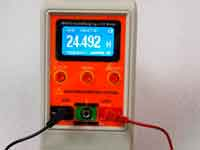Pot core inductor calculations - 3.5 out of 5 based on 2 votes
Rating 3.50 (2 Votes)

Pot core inductor calculationsFerrite cores for inductors have a wide variety species. W-shaped, U-shaped, pot cores of various modifications.  Such cores are also used with the powder iron materials. Calculation of the coils with any ferromagnetic core uses method calculation with a special parameter AL - inductance factor of the core.

The basic calculation formula:• L -  inductance (nH)
• AL - inductance factor of the core (nH/turns square)
• N - number of turns of the coil

The parameter AL can be found in the manufacturer specifications for each species of core. The main difference between pot core from the ring core is that although almost all the magnetic flux is also concentrated within the core, however, the path of the magnetic flux have a gap at the fit of the cups to each other. This gap has a high magnetic resistance and, eventually, the effective magnetic permeability of the core is always lower than the initial one.• μe - effective magnetic permeability of the core;
• μi - initial magnetic permeability;
• lg,le  - length of the gap and the average length of the magnetic flux in the core, respectively.

In addition to the gap between the cups, the value of which depends on the density of their fit to each other, there is a technological gap in the central kern of the core. This gap is intended to stabilize the parameters of the core by reducing the dependence of the relative magnetic permeability on the cups fitting. The different types of cores from different manufacturers have different gap.

The value of the inductance coefficient AL depends on μe and core sizes.• Se - effective cross - sectional area of the magnetic circuit (depends only on the size of the core);
• le - average length of the magnetic field lines (depends only on the size of the core);
• α - constant correction factor (does not depend on the size of the core).

Knowing the dimensions of the core and its initial permeability, we can determine the inductance factor of the core  AL. Because the size of the gap between the cups of a lot less than the average length of the magnetic line and the relationships between them at different sizes of cups are close - you can simplify the calculation and to operate with the initial magnetic permeability instead relative.the β - coefficient is less than one, taking into account the gap in the core.

• Ultimately, by combining the coefficients α and β in common factor k we arrive at the following empirical formulas:• L - inductance (µh)
• µi -  initial magnetic permeability of the core
• N - number of turns of the coil
• k = 19.74 for ferrite cores
• k = 60 for iron powder cores
• D1,D2,d1,d2,h1,h2 - the geometrical dimensions of the core in mm.

The average ratio of the gap to the length of the magnetic field line for the ferrite and carbonyl cores is different, and that is reflected in different values of the k-factor.

One must keep in mind that the simplification of the calculation without taking into account the real size of the magnetic gap (the introduction of β coefficient) significantly reduces the accuracy of the calculation. Additionally, the dispersion of initial µ of the core is quite high and depends on the temperature. Therefore, the calculation of the coils in pot core by this method has a considerable error of up to ±30% and above and can be useful only for cores with unknown AL. For more accurate calculations it is necessary to use the coefficient AL, if you found it in the pot core Datasheet.

Сomments from anonymous guests are enabled with moderation.

Coil Winding MachineUS \$187.53
The system consists of individual components including a controller, a stepping motor, a foot switch, power adapter and output shaft. This design makes it very easy for you to integrate the system into your own project.

LC Meter LC200AUS \$37.99
Measuring Capacitance Range:
0.01 pF - 100mF
Measuring Inductance Range:
0.001 uH - 100H
Measurement accuracy:1%.

RF InductorsUS \$6.43
Variable Ferrite Core Inductor coil set 3.5Turns 25-100MHZ

Auto Ranging LCR MeterUS \$63.07
Meter can measure 0.00pF to 100.00mF capacitance, 0.000uH to 100.00H inductance and 0.00? to 10.000M? resistance. Very suitable for small value capacitor and inductor measurement.